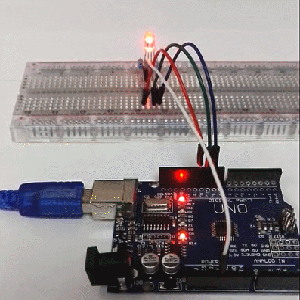To star Arduino project, RGB LED is a good way for beginners to start with. RGB LEDs just like three LEDs in one but they have 4 pins.So,just like regular LEDs,it is not difficult to use and connect them.And it is also fun and cool to add some other colors to your project.

What is RGB LED?

First of all,I think it is necessary for us to know what RGB LED is. RGB LED comes mostly in 2 versions:Common Anode and Common Cathode.Common Anode uses 5V on the common pin while common cathode connects to ground.The RGB LED has four leads.In our sketch,we use Common Anode RGB LED.The common positive connection of the led package is the second pin from the flat side.It is also the longest of the four leads and will be connected to the 5V.

Each LED inside the package requires its own 220 ohm resistor to prevent too much current flowing through it.

Why do it can show different colors?

RGB LEDs look like mostly regular LEDs,however there are actually three LEDs, one red ,one green and one blue.By controlling the brightness of each of the individual LEDs you can mix pretty colors you want.

We mix colors the same way you would mix paint on palette-by adjusting the brightness of each of the the three LEDs. If we set the brightness of all three LEDs to be the same then the overall color of the light will be white.if we turn off the blue led o that just the red and green leds are the same brightness ,then the light will appear yellow.We can control the brightness of each of the red,green and blue parts of the LEDs separately,making it possible to mix any color we like.Components

You need these components when you start this project:

Arduino UNO board *1

RGB led *1

220 ohm resistors *3

M-M wires(Male to male jumper wires)*5

USB cable *1

Connecting the hardware

1.connect the RGB LED

You should note that the anode ‘s postion because it is related to the code and the wire connection.

We connect the four pins to the breadboard in a row.

2.Connect the 220 ohm resistors

Resistors only connect to the led pins that is Red pin,Green pin and Blue pin.The common anode don’t connect to the resistor,we connect it to the 5V.

You need to connect the LED pins with their respective resistors in a column.

3.Connect the wires.3.Connect the wires

We connect a jump wire after the Red resistor,Green resistor and Blue resistor.And a long jump wire connect to the anode pin.

We use red wire to connect Red LED pin ,green wire to Green Led pin ,blue wire to Blue pin and White wire to anode pin.

And then,connect the red wire to Arduino UNO board pin11,green wire to pin10,blue wire to pin9 and white wire to 5V pin.Till now,we have finish all the connection of hardware!

We open the arduino IDE and begin to write code for this RGB LED.But before writing the code,we should consider these things:

1.define the led pin,

2.define the pinMode status;

3.set color;

4.set the delay time.

As for the question 1,2 &4,we have learned code editing in the previous chapters.

Then,how to set color of the RGB LED using the code?Here,we use

setColor(PWM1, PWM2, PWM3);

The PWM changes from 0 to 255 so the RGB LED can change many colors.

When the PWM1=255,PWM2= 0,PWM3= 0,the RGB LED turns to be red.When the PWM1=0,PWM2= 255,PWM3= 0,the RGB LED turns to be green.When the PWM1=0,PWM2= 0,PWM3= 255,the RGB LED turns to be bule. When the PWM1=255,PWM2= 255,PWM3= 0,the RGB LED turns to be yellow.When the PWM1=255,PWM2= 0,PWM3= 255,the RGB LED turns to be purple.

Knowing about it ,so we can write our code like this:

int redPin = 11;

int greenPin = 10;

int bluePin = 9;

void setup()

{

pinMode(redPin, OUTPUT);

pinMode(greenPin, OUTPUT);

pinMode(bluePin, OUTPUT);

}

void loop()

{

setColor(255, 0, 0);  // set color red

delay(500);

setColor(0, 250, 0);  // set color green

delay(500);

setColor(0, 0, 255);  // set color blue

delay(500);

setColor(255, 0, 255);  // set color purple

delay(500);

setColor(255, 255, 0);  // set color yellow

delay(500);

}

void setColor(int red, int green, int blue)

{

analogWrite(redPin, 255-red);

analogWrite(greenPin, 255-green);

analogWrite(bluePin, 255-blue);

}

And then,we upload the code to Arduino R3 board through Arduino IDE.When we finish these,our RGB LED will change colors like this: